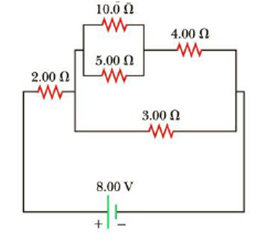Chapter 18, Problem 10P

Chapter
Section
Textbook Problem

Consider the circuit shown in Figure P18.10. (a) Calculate the equivalent resistance of the 10.0-Ω and 5.00-Ω resistors connected in parallel. (b) Using the result of part (a), calculate the combined resistance of the 10.0-Ω, 5.00-Ω, and 4.00-Ω resistors. (c) Calculate the equivalent resistance of the combined resistance found in part (b) and the parallel 3.00-Ω resistor. (d) Combine the equivalent resistance found in part (c) with the 2.00-Ω resistor. (e) Calculate the total current in the circuit. (f) What is the voltage drop across the 2.00-Ω resistor? (g) Subtracting the result of part (f) from the battery voltage, find the voltage across the 3.00-Ω resistor. (h) Calculate the current in the 3.00-Ω resistor.Figure P18.10

(a)

To determine
The equivalent resistance of the first parallel combination of resistors.

Explanation

Given Info: The resistors connected in parallel combination is 10.0Ω and 5.00Ω .

Explanation:

Formula to calculate the equivalent resistance of the first parallel combination resistors is,

1Rp1=1R1+1R2

• Rp1 is the equivalent resistance of parallel combination resistor,
• R1 and R2 is the resistors,

Substitute 10.0Ω for R1 and 5

(b)

To determine
The combined resistance of the resistors in series combination using the result in part (a).

(c)

To determine
The equivalent resistance of the second parallel combination of resistors shown in the diagram.

(d)

To determine
The combined resistance of the resistors in second series combination for the entire circuit.

(e)

To determine
The total current passing through the circuit.

(f)

To determine
The potential drop across the 2.00Ω resistor.

(g)

To determine
The potential drop across the second parallel combination resistance.

(h)

To determine
The current passing through the 3.00Ω resistor in the circuit.

Still sussing out bartleby?

Check out a sample textbook solution.

See a sample solution

The Solution to Your Study Problems

Bartleby provides explanations to thousands of textbook problems written by our experts, many with advanced degrees!

Get Started

Find more solutions based on key concepts